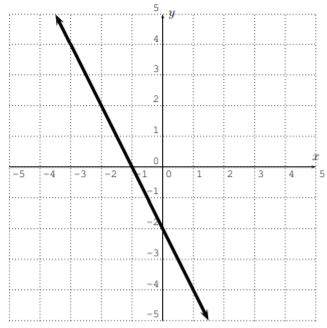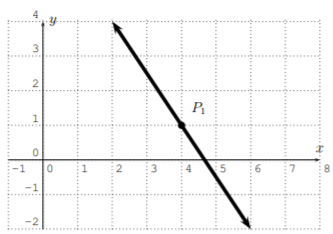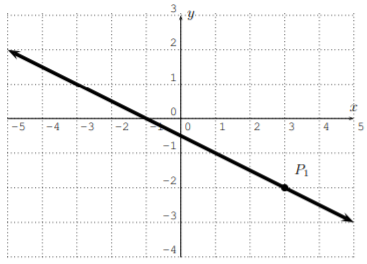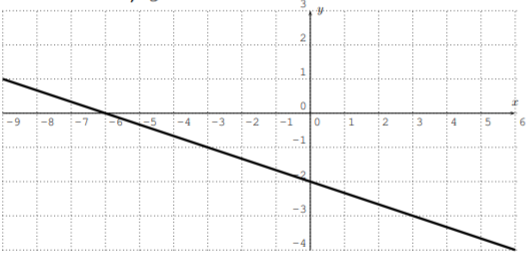# 2.3: Exercises

$$\newcommand{\vecs}{\overset { \rightharpoonup} {\mathbf{#1}} }$$ $$\newcommand{\vecd}{\overset{-\!-\!\rightharpoonup}{\vphantom{a}\smash {#1}}}$$$$\newcommand{\id}{\mathrm{id}}$$ $$\newcommand{\Span}{\mathrm{span}}$$ $$\newcommand{\kernel}{\mathrm{null}\,}$$ $$\newcommand{\range}{\mathrm{range}\,}$$ $$\newcommand{\RealPart}{\mathrm{Re}}$$ $$\newcommand{\ImaginaryPart}{\mathrm{Im}}$$ $$\newcommand{\Argument}{\mathrm{Arg}}$$ $$\newcommand{\norm}{\| #1 \|}$$ $$\newcommand{\inner}{\langle #1, #2 \rangle}$$ $$\newcommand{\Span}{\mathrm{span}}$$ $$\newcommand{\id}{\mathrm{id}}$$ $$\newcommand{\Span}{\mathrm{span}}$$ $$\newcommand{\kernel}{\mathrm{null}\,}$$ $$\newcommand{\range}{\mathrm{range}\,}$$ $$\newcommand{\RealPart}{\mathrm{Re}}$$ $$\newcommand{\ImaginaryPart}{\mathrm{Im}}$$ $$\newcommand{\Argument}{\mathrm{Arg}}$$ $$\newcommand{\norm}{\| #1 \|}$$ $$\newcommand{\inner}{\langle #1, #2 \rangle}$$ $$\newcommand{\Span}{\mathrm{span}}$$$$\newcommand{\AA}{\unicode[.8,0]{x212B}}$$

## Exercise $$\PageIndex{1}$$

Find the slope and $$y$$-intercept of the line with the given data. Using the slope and $$y$$-intercept, write the equation of the line in slope-intercept form.

1.2.3.4.5.6.1. $$y=2 x-4$$
2. $$y=-x+3$$
3. $$y=-2 x-2$$
4. $$y=\dfrac{2}{5} x+3$$
5. $$y=-x+0$$ or$$y=-x$$
6. $$y=\dfrac{2}{3} x+4$$

## Exercise $$\PageIndex{2}$$

Write the equation of the line in slope-intercept form. Identify slope and $$y$$-intercept of the line.

1. $$4x+2y=8$$
2. $$9x-3y+15=0$$
3. $$-5x-10y=20$$
4. $$3x-5y=7$$
5. $$-12x+8y=-60$$
6. $$8x-9y=0$$
1. $$y=-2 x+4$$
2. $$y=3 x+5$$
3. $$y=-\dfrac{1}{2} x-2$$
4. $$y=\dfrac{3}{5} x-\dfrac{7}{5}$$
5. $$y=\dfrac{3}{2} x-\dfrac{15}{2}$$
6. $$y=\dfrac{8}{9} x$$

## Exercise $$\PageIndex{3}$$

Find the equation of the line in point-slope form (Eq. 2.1.2) using the indicated point $$P_1$$.

1.2.3.4.1. $$y-3=\dfrac{1}{3} \cdot(x-5)$$
2. $$y-1=-\dfrac{3}{2} \cdot(x-4)$$
3. $$y+2=-\dfrac{1}{2} \cdot(x-3)$$
4. $$y-1=1 \cdot(x+1)$$

## Exercise $$\PageIndex{4}$$

Graph the line by calculating a table (as in Example 2.1.1). (Solve for $$y$$ first, if this is necessary.)

1. $$y=2x-4$$
2. $$y=-x+4$$
3. $$y=\dfrac 1 2 x +1$$
4. $$y=3x$$
5. $$8x-4y=12$$
6. $$x+3y+6=0$$
1.2.3.4.5. $$y=2 x-3$$6. $$y=-\dfrac{1}{3} x-2$$## Exercise $$\PageIndex{5}$$

Determine if the given table describes a function. If so, determine its domain and range. Describe which outputs are assigned to which inputs.

1. $\begin{array}{|c||c|c|c|c|c|} \hline x & -5 & 3 & -1 & 6 & 0 \\ \hline \hline y & 5 & 2 & 8 & 3 & 7 \\ \hline \end{array} \nonumber$
2. $\begin{array}{|c||c|c|c|c|c|} \hline x & 6 & 17 & 4 & -2 & 4 \\ \hline \hline y & 8 & -2 & 0 & 3 & -1 \\ \hline \end{array} \nonumber$
3. $\begin{array}{|c||c|c|c|c|c|c|} \hline x & 19 & 7 & 6 & -2 & 3 & -11 \\ \hline \hline y & 3 & 3 & 3 & 3 & 3 & 3 \\ \hline \end{array} \nonumber$
4. $\begin{array}{|c||c|c|c|c|c|c|} \hline x & 1 & 2 & 3 & 3 & 4 & 5 \\ \hline \hline y & 5.33 & 9 & 13 & 13 & 17 & \sqrt{19} \\ \hline \end{array} \nonumber$
5. $\begin{array}{|c||c|c|c|c|c|c|} \hline x & 0 & 1 & 2 & 2 & 3 & 4 \\ \hline \hline y & 0 & 1 & 2 & 3 & 3 & 4 \\ \hline \end{array} \nonumber$
1. this is a function with domain $$D=\{-5,-1,0,3,6\}$$ and range $$R = \{2, 3, 5, 7, 8\}$$, for example: the input $$x = −5$$ gives output $$y = 5$$, etc.
2. not a function since for $$x = 4$$ we have both $$y = 0$$ and $$y = −1$$
3. this is a function with $$D = \{−11, −2, 3, 6, 7, 19\}$$, $$R = \{3\}$$
4. this is a function with $$D=\{1,2,3,4,5\}, R=\{\sqrt{19}, 5.33,9,13,17\}$$
5. this is not a function

## Exercise $$\PageIndex{6}$$

We consider children and their (birth) mothers.

1. Does the assignment child to their birth mother constitute a function (in the sense of Definition as stated here)?
2. Does the assignment mother to their children constitute a function?
3. In the case where the assignment is a function, what is the domain?
4. In the case where the assignment is a function, what is the range?
1. yes
2. no
3. the domain for the function in (a) is the set of all children
4. the range for the function in (a) is the set of all mothers

## Exercise $$\PageIndex{7}$$

A bank offers wealthy customers a certain amount of interest, if they keep more than $$1$$ million dollars in their account. The amount is described in the following table:

$\begin{array}{|c||c|} \hline \text { dollar amount } x \text { in the account } & \text { interest amount } \\ \hline \hline x \leq \ 1,000,000 & \ 0 \\ \hline \ 1,000,000<x \leq \ 10,000,000 & 2 \% \text { of } x \\ \hline \ 10,000,000<x & 1 \% \text { of } x \\ \hline \end{array} \nonumber$

1. Justify that the assignment cash amount to interest defines a function.
2. Find the interest for an amount of:
1. $$\50,000$$
2. $$\5,000,000$$
3. $$\1,000,000$$
4. $$\30,000,000$$
5. $$\10,000,000$$
6. $$\2,000,000$$
1. a given cash amount $$x$$ determines the interest amount $$y$$
2. i) $$\0$$, ii) $$\100,000$$, iii) $$\0$$, iv) $$\300,000$$, v) $$\200,000$$, vi) $$\40,000$$

## Exercise $$\PageIndex{8}$$

Find a formula for a function describing the given inputs and outputs.

1. input: the radius of a circle, output: the circumference of the circle
2. input: the side length in an equilateral triangle, output: the perimeter of the triangle
3. input: one side length of a rectangle, with other side length being $$3$$, output: the perimeter of the rectangle
4. input: the side length of a cube, output: the volume of the cube
1. $$C=2 \pi r$$
2. $$P=3 a$$
3. $$P=2 a+6$$
4. $$V=a^{3}$$